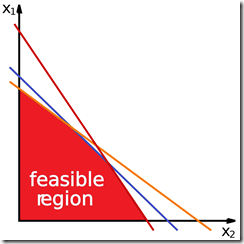Want to share your content on R-bloggers? click here if you have a blog, or here if you don't.

Linear programming is a technique to solve optimization problems whose constraints and outcome are represented by linear relationships.Simply put, linear programming allows to solve problems of the following kind:

• Maximize/minimize $\hat C^T \hat X$
• Under the constraint $\hat A \hat X \leq \hat B$
• And the constraint $\hat X \geq 0$

This doesn’t seem much when you glance at it but in practice it is a powerful tool that can be used to make decisions in practical life scenarios.

It is often the case that we need to make decisions based on constraints. Often the invisible and most harsh constraint is time, but generally speaking there are a lot of other constraints that we need to take into account. A simple set of examples would be:

• I want to change my heating system. I want to minimize the cost of the system and the bills, what kind of heating system should I install? A pellet stove? Electric radiators?
• I want to obtain the maximum profit from the sale of these two products I produce. In what quantities should I produce each product?
• And so on…

1. The function you are trying to optimize is a linear combination of the decision variables (this might not always be the case).
2. The constraints you have are a linear combination of the decision variables.

## An example of linear optimization

I’m going to implement in R an example of linear optimization that I found in the book “Modeling and Solving Linear Programming with R” by Jose M. Sallan, Oriol Lordan and Vincenc Fernandez.  The example is named “Production of two models of chairs” and can be found at page 57, section 3.5. I’m going to solve only the first point.

The problem text is the following

A company produces two models of chairs: 4P and 3P. The model 4P needs 4 legs, 1 seat and 1 back. On the other hand, the model 3P needs 3 legs and 1 seat. The company has a initial stock of 200 legs, 500 seats and 100 backs. If the company needs more legs, seats and backs, it can buy standard wood blocks, whose cost is 80 euro per block. The company can produce 10 seats, 20 legs and 2 backs from a standard wood block. The cost of producing the model 4P is 30 euro/chair, meanwhile the cost of the model 3P is 40 euro/chair. Finally, the company informs that the minimum number of chairs to produce is 1000 units per month. Define a linear programming model, which minimizes the total cost (the production costs of the two chairs, plus the buying of new wood blocks).

## Problem definition

First, we need to translate the problem in a mathematical way. Let’s define the following variables

• $x_{4p}$ is the number of 4P chairs to be produced.
• $x_{3p}$ is the number of 3P chairs to be produced.
• $x_w$ is the number of wood blocks to be bought.

Now we can define $\hat X = \begin{pmatrix} x_{4p} \\ x_{3p} \\ x_w \end{pmatrix}$ as the decision variable vector. Note that it must be $\hat X \geq 0$.

We would like to minimize the total cost so we must set our objective function as follows

$$cost(x_{4p}, x_{3p}, x_w) = 30 x_{4p} + 40 x_{3p} + 80 x_w = MIN(cost)$$

which means that $\hat C = \begin{pmatrix} 30 \\ 40 \\ 80 \end{pmatrix}$.

The constraints can be set in the following way

1. For the seats $$x_{4p} + x_{3p} \leq 500 + 10 x_w$$
2. For the legs $$4 x_{4p} + 3 x_{3p} \leq 200 + 20 x_w$$
3. For the backs $$x_{4p} \leq 100 + 2 x_w$$
4. Minimum number of chairs produced $$x_{4p} + x_{3p} \geq 1000$$

We can now define the coefficient matrix $A = \begin{pmatrix} 1 & 1 & -10 & \\ 4 & 3 & -20 & \\ 1 & 0 & -2 & \\ – 1 & – 1 & 0 & \end{pmatrix}$ and $B = \begin{pmatrix} 500 \\ 200 \\ 100 \\ -1000 \end{pmatrix}$.

## R implementation and solution

We are now ready to implement this is in R. There are many different packages which can solve this kind of problems but my favourites are lpSolve and lpSolveAPI which is a kind of API built on top of lpSolve. I will use both.

A nice feature about the lpSolve package is that you can specify the direction of the constraints. Indeed in our case the last constraint of minimum number of chairs produced does not fit in with the mathematical definiton of the problem that we gave in the previous paragraph. Here we can either change the signs (and therefore the inequality direction) or specify the inequality direction in lpSolve. I’ll do this second way.

We can set that all the variables should be integers by setting the argument “all.int=TRUE” in the lpSolve::lp function. Of course, lpSolve can work with both integers and real numbers.

It’s also particularly important to check the status at the end of the execution: if it is 0, then a solution has been found but if it is 2 then this means that there is no feasible solution.